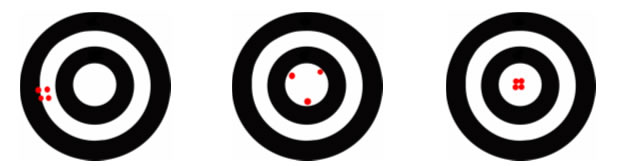# Difference between Accuracy and Precision

##### Key Difference: Accuracy is the degree of closeness of a measurement of a quantity to a “true” value, while precision is the number of times a measurement is close to a central value.

Accuracy and precision are most commonly used in fields such as science, engineering, industry and statistics. Accuracy and precision are synonymous; however they are very different from each other. Accuracy is the degree of closeness of a measurement of a quantity to a “true” value. Precision is the number of times a measurement is close to a central value.

Accuracy is the amount of times a person is close to the ‘actual’ or ‘true’ value, while precision is the amount of times a person comes up with results similar to a value, which may or may not be precise. A measurement can be accurate, precise, both or neither. However, if a value is precise does not necessarily mean that the value is accurate, however if a person keeps coming up with accurate results each time, it means the results are accurate and precise.

Let’s take accuracy and precision in terms of a target:In the first image, the person shooting the arrow or the ‘results’ are precise but not accurate. The image in the middle shows that the shooter is accurate as the points are in the bullseye, but the person is not precise as the points are not closer to each other. The last image on the right shows that the shooter is accurate as well as precise.

In terms of language, accurate is described as truth or without any errors, while precise is described as defined, strict, neither more nor less.